## Kinetic theory

12-1-99

Sections 13.11 - 13.15

We're now going to draw on much of what we've learned in this course to understand the motion of gases at the molecular level. We'll examine the ideal gas law from the perspective of physics, and we'll come to a deeper understanding of what temperature is.

### Kinetic theory

Consider a cube-shaped box, each side of length L, filled with molecules of an ideal gas. A molecule of ideal gas is like a bouncy rubber ball; whenever it's involved in a collision with a wall of the box, it rebounds with the same kinetic energy it had before hitting the wall. Similarly, if ideal gas molecules collide, the collisions are elastic, so no kinetic energy is lost.

Now consider one of these ideal gas molecules in this box, with a mass m and velocity v. If this molecule bounces off one of the walls perpendicular to the x-direction, the y and z components of the molecule's velocity are unaffected, and the x-component of velocity reverses. The molecule maintains the same speed, because the collsion is elastic. How much force, on average, does it exert on the wall?

To answer this we just have to think about momentum, and impulse. Momentum is a vector, so if the particle reverses its x-component of momentum there is a net change in momentum of 2 m vx. The magnitude of the average force exerted by the wall to produce this change in momentum can be found from the impulse equation: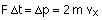If we're dealing with the average force, the time interval is just the time between collisions, which is the time it takes for the molecule to make a round trip in the box and come back to hit the same wall. This time simply depends on the distance travelled in the x-direction (2L), and the x-component of velocity; the time between collisions with that wall is 2L / vx.

Solving for the average force gives: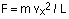This is the magnitude of the average force exerted by the wall on the molecule, as well as the magnitude of the average force exerted by the molecule on the wall; the forces are equal and opposite.

This is just the average force exerted on one wall by one molecule selected at random. To find the total force, we need to include all the other molecules, which travel at a wide range of speeds. The distribution of speeds follows a curve that looks a lot like a Bell curve, with the peak of the distribution increasing as the temperature increases. The shape of the speed distribution is known as a Maxwellian distribution curve, named after James Clerk Maxwell, the Scottish physicist who first worked it out.

To find the total force on the wall, then, we can just add up all the individual forces:Multiplying and dividing the right-hand side by N, the number of molecules in the box, gives: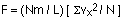The term in square brackets is simply an average...we're adding up the square of the x-component of velocity for all the molecules and dividing by the number of molecules. This average is known as the root-mean-square average, symbolized by rms.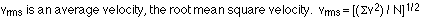Therefore: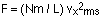Consider now how this average x velocity compares to the average velocity. For any molecule, the velocity can be found from its components using the Pythagorean theorem in three dimensions:For a randomly-chosen molecule, the x, y, and z components of velocity may be similar but they don't have to be. If we take an average over all the molecules in the box, though, then the average x, y, and z speeds should be equal, because there's no reason for one direction to be preferred over another. So:The force exerted by the molecules on a wall of the box can then be expressed in terms of the average velocity, rather than the average x component of velocity.The pressure exerted by the gas on each wall is simply the force divided by the area of a wall. Therefore: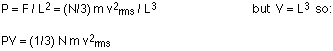Rearranging things a little gives: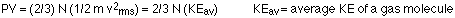This equation has many of the same variables as the ideal gas law, PV = NkT. This means that: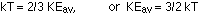This is a very important result, because it tells us something fundamental about temperature. The absolute temperature of an ideal gas is proportional to the average kinetic energy per gas molecule. If changes in pressure and/or volume result in changes in temperature, it means the average kinetic energy of the molecules has been changed.

### The internal energy of an ideal gas

The result above says that the average translational kinetic energy of a molecule in an ideal gas is 3/2 kT. For a gas made up of single atoms (the gas is monatomic, in other words), the translational kinetic energy is also the total internal energy. Rotational kinetic energy can be ignored, because the atoms are so small that the moment of inertia is negligible.There is also no energy associated with bonds between atoms in molecules, because there are no bonds in a monatomic gas. For a monatomic ideal gas, then, the total internal energy (U) is simply: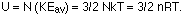Note that gases made up of molecules with more than one atom per molecule have more internal energy than 3/2 nRT, because energy is associated with the bonds and the vibration of the molecules. Another interesting result involves the equipartition of energy, which says that each contributer to the internal energy contributes an equal amount of energy. For a monatomic ideal gas, each of the three directions (x, y, and z) contribute 1/2 kT per molecule, for a total of 3/2 kT per molecule.

For a diatomic molecule, the three translation direction contribute 1/2 kT per molecule, and for each molecule there are also two axes of rotation, contributing rotational kinetic energy in the amount of 1/2 kT each. This amounts to a total internal energy of U = 5/2 NkT for a diatomic gas, and a polyatomic gas has contributions from three translation direction and three axes of rotation, giving U = 6/2 NkT, or 3NkT.

### Diffusion

Physics is full of cases where similar behavior in different kinds of systems can be described by similar equations. Diffusion, for example, the flow of a substance from a region of higher concentration to a region of lower concentration, is a similar process to thermal conductivity, which involves heat flowing from a higher temperature region to a lower temperature region. The similarities can be seen in the equations: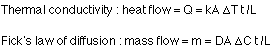Both equations involve a length, a time, a cross-sectional area, and a constant. D is the diffusion constant, while k is the thermal conductivity; both depend on what the flow is passing through. In addition, both equations involve differences; temperature difference for heat flow, and a concentration difference in the case of the mass flow.

Always look for parallels. If you can relate one system back to another system you already understand, you'll be able to figure out the new system much more easily.